# 13.5. Speedgoat QNX¶

Important

When deploying to a target hardware platform, the library included in the lib_target directory of the generated solver should be used instead of the library in the lib directory.

## 13.5.1. High-level interface¶

### 13.5.1.1. Instructions¶

The steps to deploy and simulate a FORCESPRO controller on a Speedgoat QNX platform are detailed below.

1. (Figure 13.87) Set the code generation options:

codeoptions.platform = 'Speedgoat-QNX'; % to specify the platform
codeoptions.printlevel = 0; % on some platforms printing is not supported
codeoptions.cleanup = 0; % to keep necessary files for target compile


and then generate the code for your solver (henceforth referred to as “FORCESNLPsolver”, placed in the folder “BasicExample”) using the high-level interface.

1. (Figure 13.88) Create a new Simulink model using the blank model template.

2. (Figure 13.89) Populate the Simulink model with the system you want to control.

3. (Figure 13.90) Make sure the FORCESNLPsolver_simulinkBlock.mexw64 file (created during code generation) is on the Matlab path.

4. (Figure 13.91) Open the FORCESNLPsolver_lib.mdl Simulink model file, contained in the interface folder of the FORCESNLPsolver folder created during code generation.

5. (Figure 13.92) Copy-paste the FORCESPRO Simulink block into your simulation model and connect its inputs and outputs appropriately.

6. (Figure 13.93) Access the Simulink Model’s Settings.

7. (Figure 13.94) In the “Solver” tab, set the options:

• Simulation start/stop time: Depending on the simulation wanted.

• Solver type: Discrete or fixed-step.

• Fixed-step size: Needs to be higher than the execution time of the solver.

1. (Figure 13.95) In the “Code Generation” tab, set the options:

• System target file: slrealtime.tlc

• Generate makefile: Off

1. (Figure 13.96) In the “Code Generation/Custom Code” tab, include the directories:

• BasicExample

• BasicExample\FORCESNLPsolver\interface

• BasicExample\FORCESNLPsolver\lib_target

1. (Figure 13.97) In the “Code Generation/Custom Code” tab, add the source files:

• FORCESNLPsolver_simulinkBlock.c

• FORCESNLPsolver_adtool2forces.c

• FORCESNLPsolver_casadi.c

1. (Figure 13.98) In the “Code Generation/Custom Code” tab, add the library file:

• libFORCESNLPsolver.a

1. (Figure 13.99) Access the FORCESPRO block’s parameters.

2. (Figure 13.100) Remove “FORCESNLPsolver” and “FORCESNLPsolver_simulinkBlock” from the S-function module.

3. (Figure 13.101) Compile the code of the Simulink model. This will also automatically load the model to the connected Speedgoat platform.

4. Deployment is complete and simulations can now be run on the Speedgoat platform.

### 13.5.1.2. Figures¶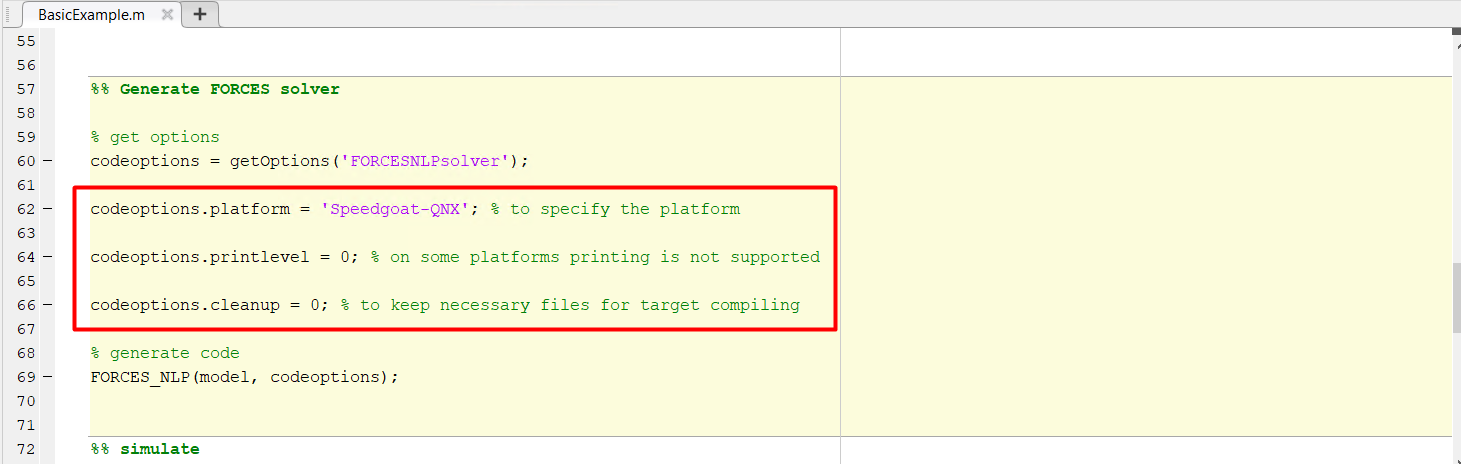Figure 13.87 Set the appropriate code generation options.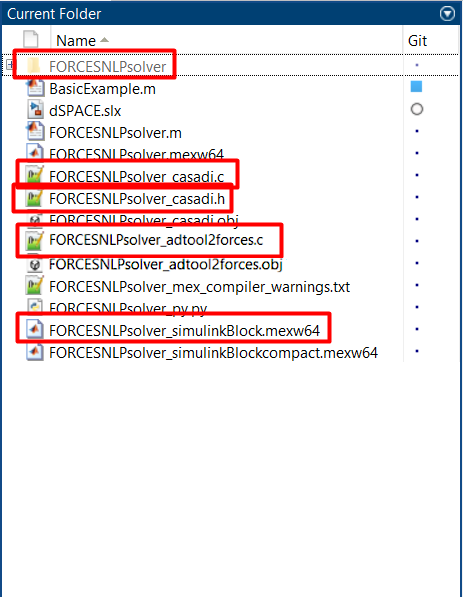Figure 13.90 Add the folder containing the .mexw64 solver file to the Matlab path.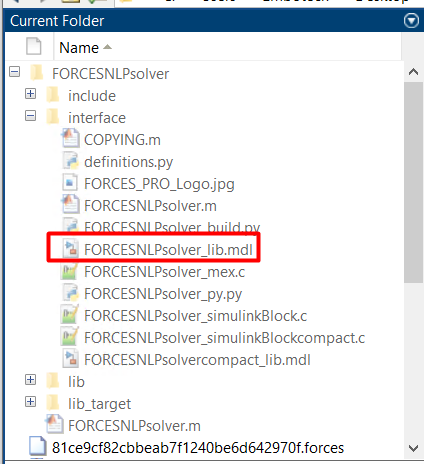Figure 13.91 Open the generated Simulink solver model.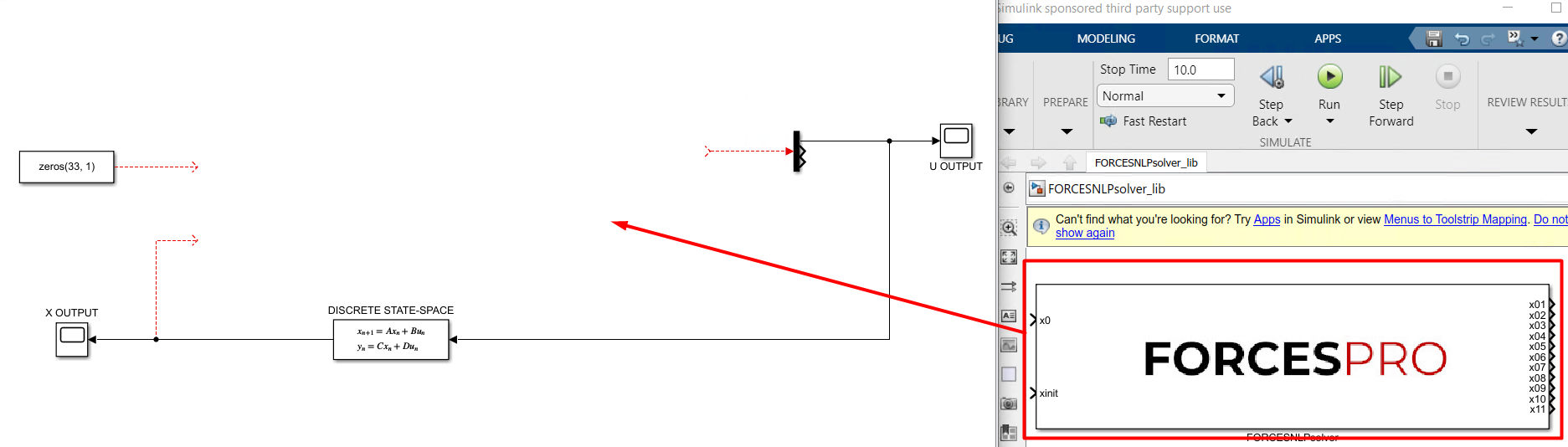Figure 13.92 Copy-paste and connect the FORCESPRO block.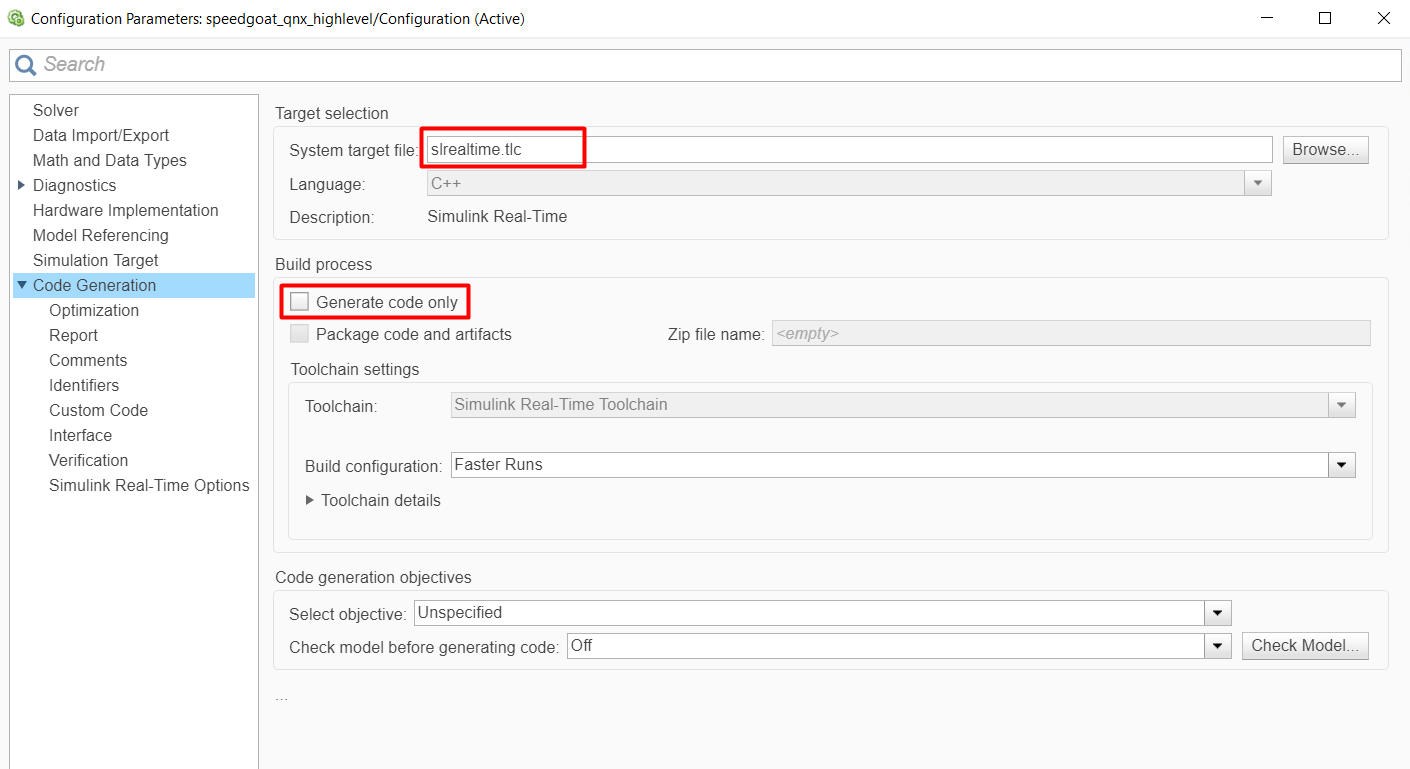Figure 13.95 Set the Simulink code generation options.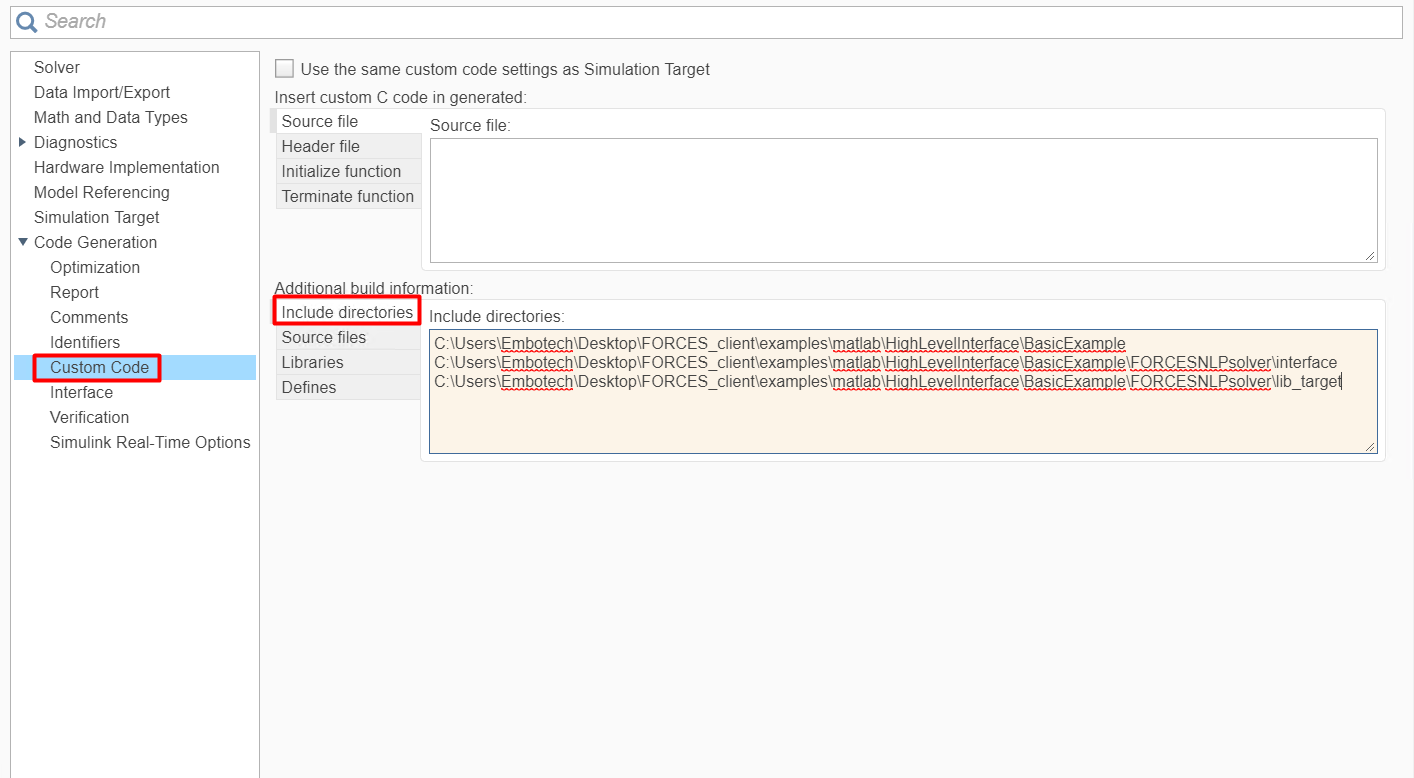Figure 13.96 Add the directories included for the code generation.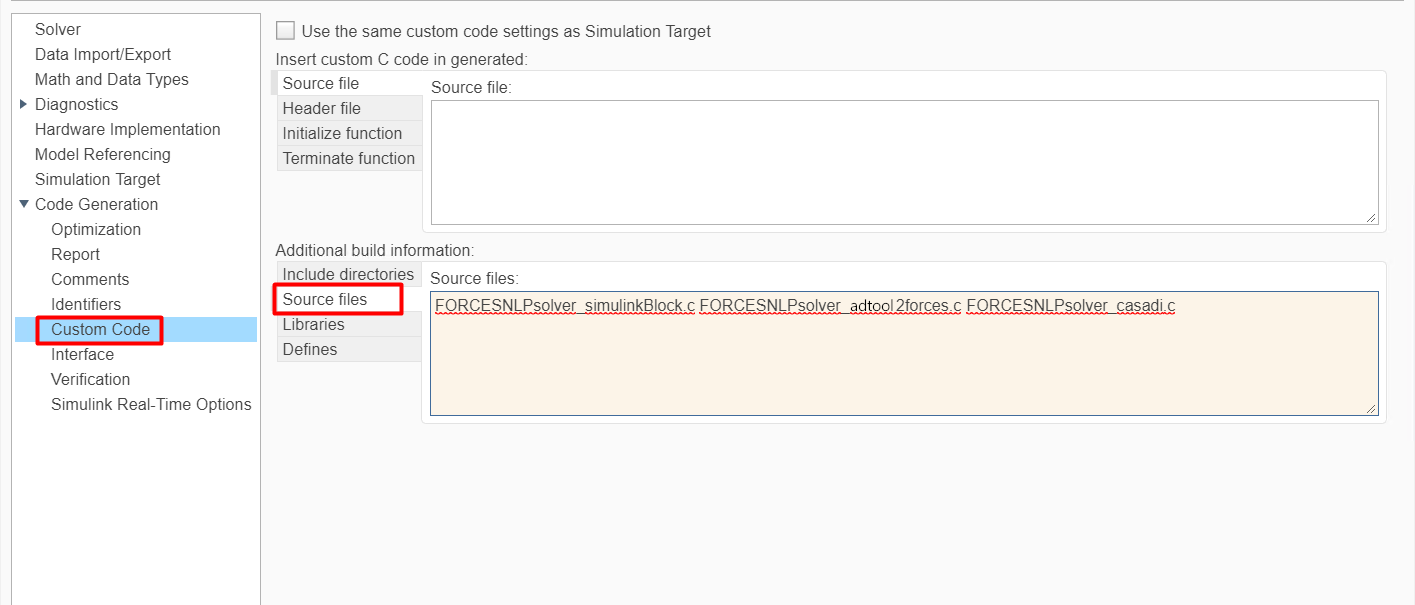Figure 13.97 Add the source files used for the code generation.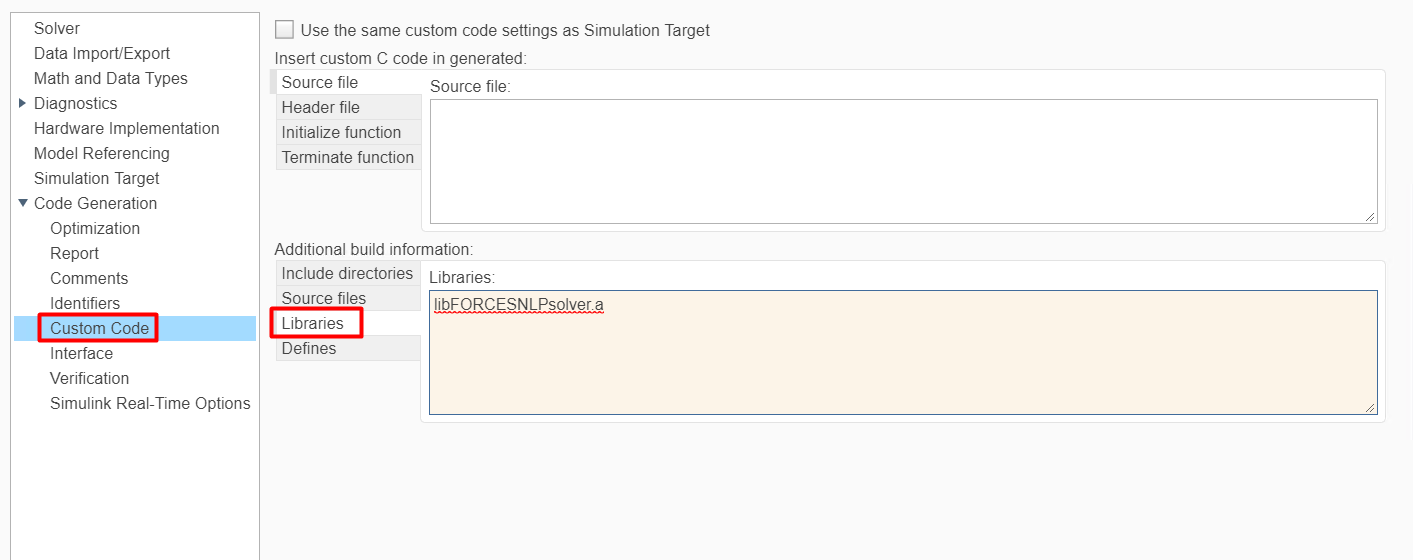Figure 13.98 Add the libraries used for the code generation.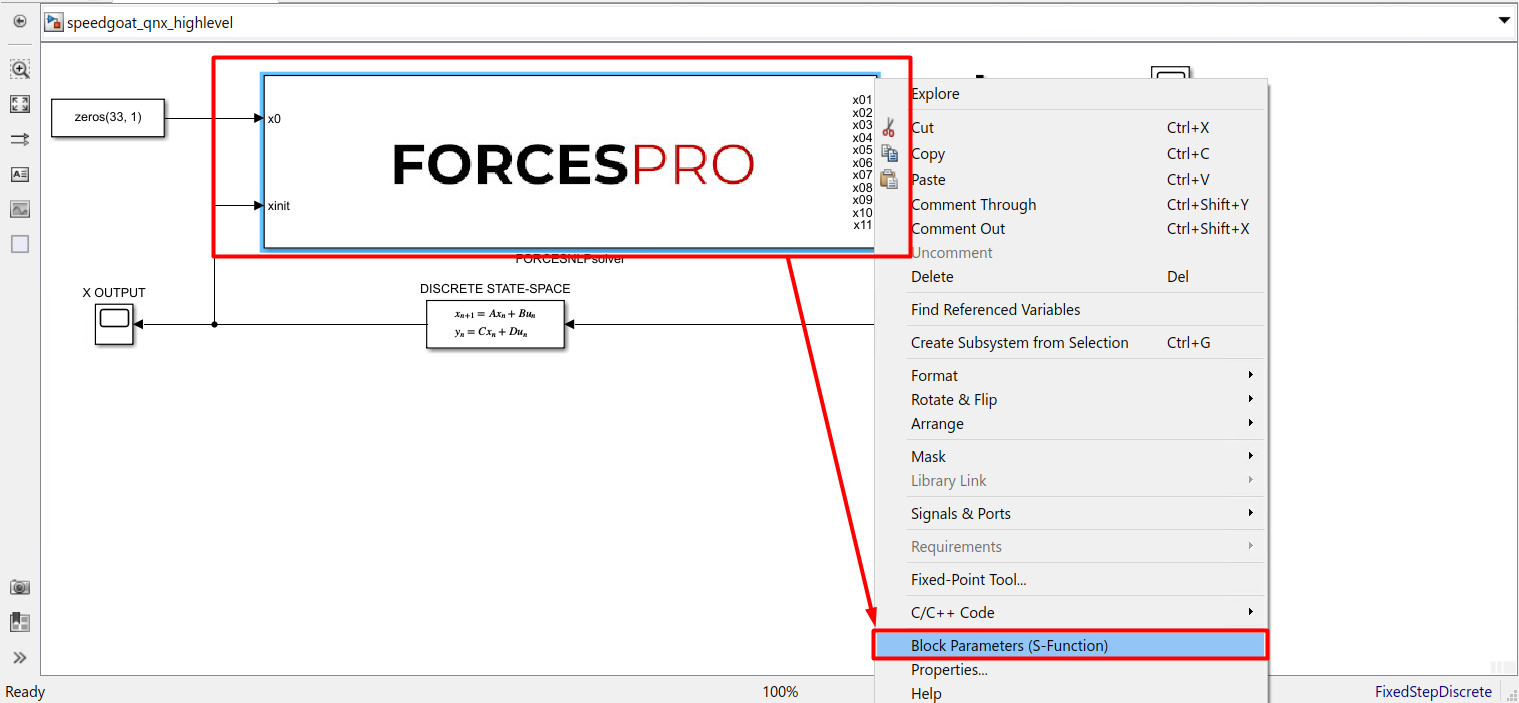Figure 13.99 Open the FORCESPRO block’s parameters.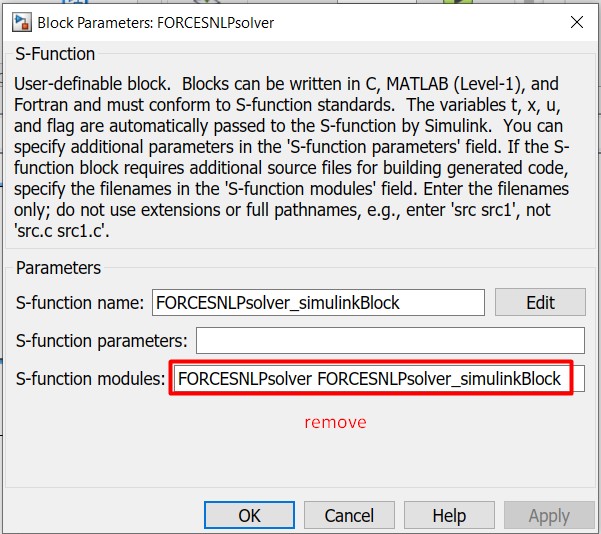Figure 13.100 Remove the default data from the S-function module.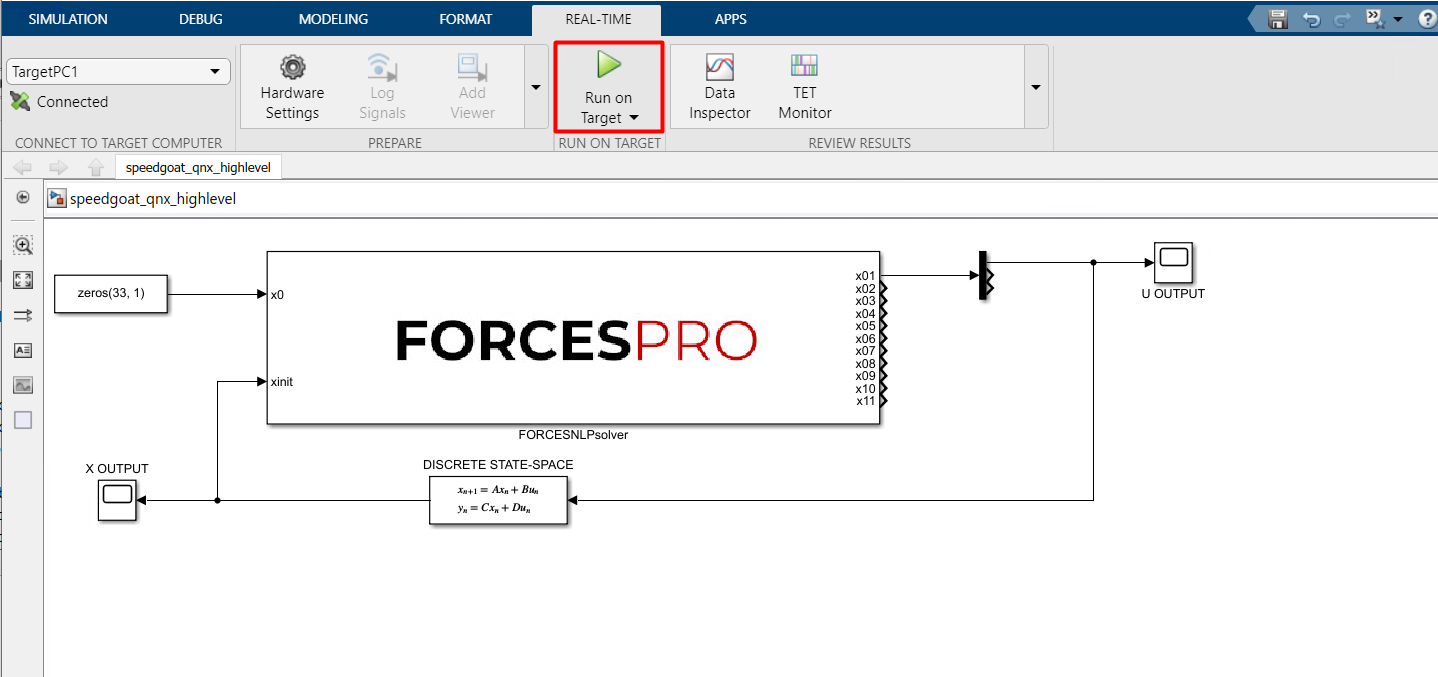Figure 13.101 Compile the code of the Simulink model.

## 13.5.2. Y2F interface¶

### 13.5.2.1. Instructions¶

The steps to deploy and simulate a FORCESPRO controller on a Speedgoat QNX platform are detailed below.

1. (Figure 13.102) Set the code generation options:

codeoptions.platform = 'Speedgoat-QNX'; % to specify the platform
codeoptions.printlevel = 0; % on some platforms printing is not supported


and then generate the code for your solver (henceforth referred to as “simplempc_solver”, placed in the folder “Y2F”) using the Y2F interface.

1. (Figure 13.103) Create a new Simulink model using the blank model template.

2. (Figure 13.104) Populate the Simulink model with the system you want to control.

3. (Figure 13.105) Make sure the simplempc_solver_simulinkBlock.mexw64 file (created during code generation) is on the Matlab path.

4. (Figure 13.106) Copy-paste the FORCESPRO Simulink block, contained in the created y2f_simulink_lib.slx Simulink model file, into your simulation model and connect its inputs and outputs appropriately.

5. (Figure 13.107) Access the Simulink Model’s Settings.

6. (Figure 13.108) In the “Solver” tab, set the options:

• Simulation start/stop time: Depending on the simulation wanted.

• Solver type: Discrete or fixed-step.

• Fixed-step size: Needs to be higher than the execution time of the solver.

1. (Figure 13.109) In the “Code Generation/RTI general build options” tab, set the options:

• System target file: slrealtime.tlc

• Generate makefile: Off

1. (Figure 13.110) In the “Code Generation/Custom Code” tab, include the directories:

• Y2F

• Y2F\simplempc_solver\interface

• Y2F\simplempc_solver\lib_target

1. (Figure 13.111) In the “Code Generation/Custom Code” tab, add the source files:

• simplempc_solver_simulinkBlock.c

• simplempc_solver.c

1. (Figure 13.112) In the “Code Generation/Custom Code” tab, add the library file:

• libinternal_simplempc_solver_1.a

1. (Figure 13.113) Compile the code of the Simulink model. This will also automatically load the model to the connected Speedgoat platform.

2. Deployment is complete and simulations can now be run on the Speedgoat platform.

### 13.5.2.2. Figures¶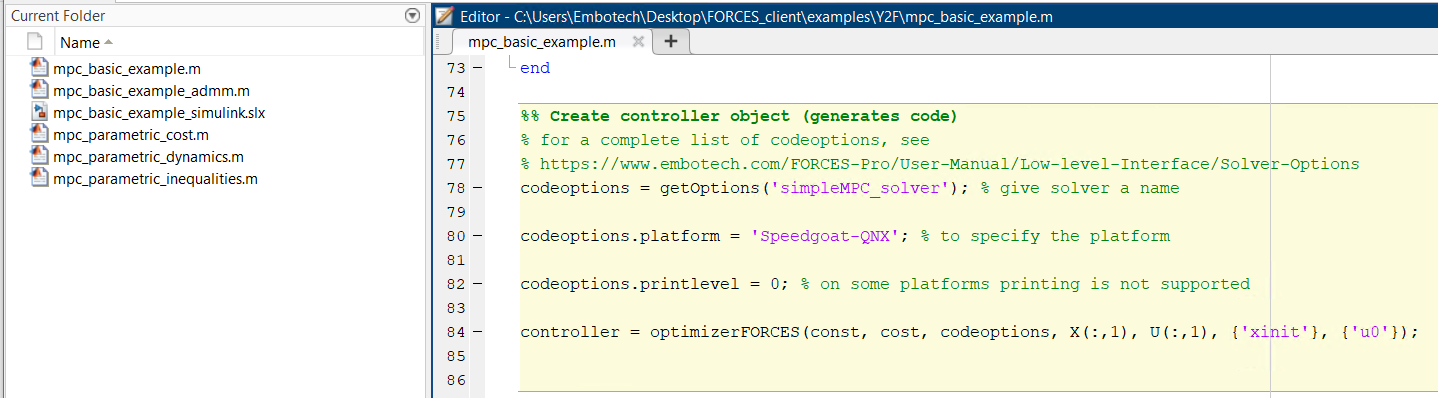Figure 13.102 Set the appropriate code generation options.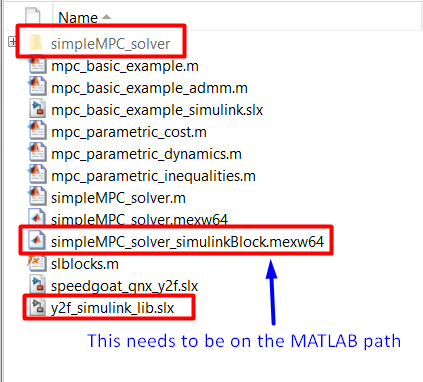Figure 13.105 Add the folder containing the .mexw64 solver file to the Matlab path.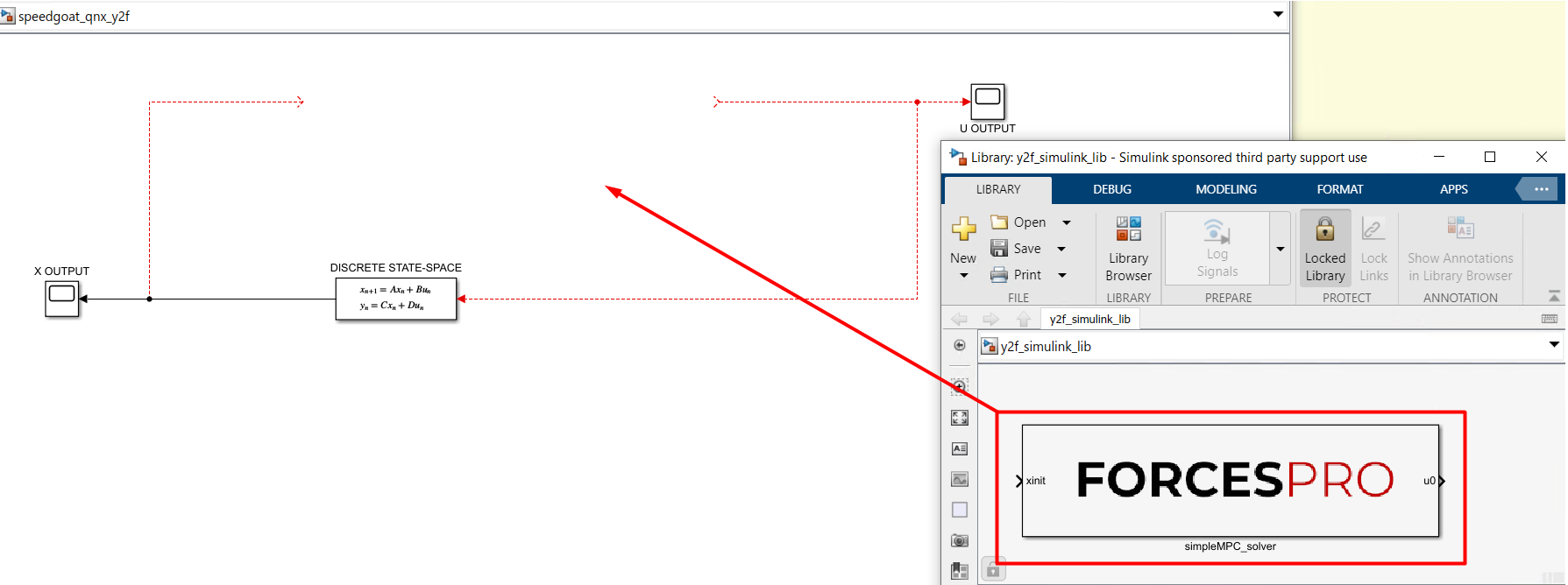Figure 13.106 Copy-paste and connect the FORCESPRO block.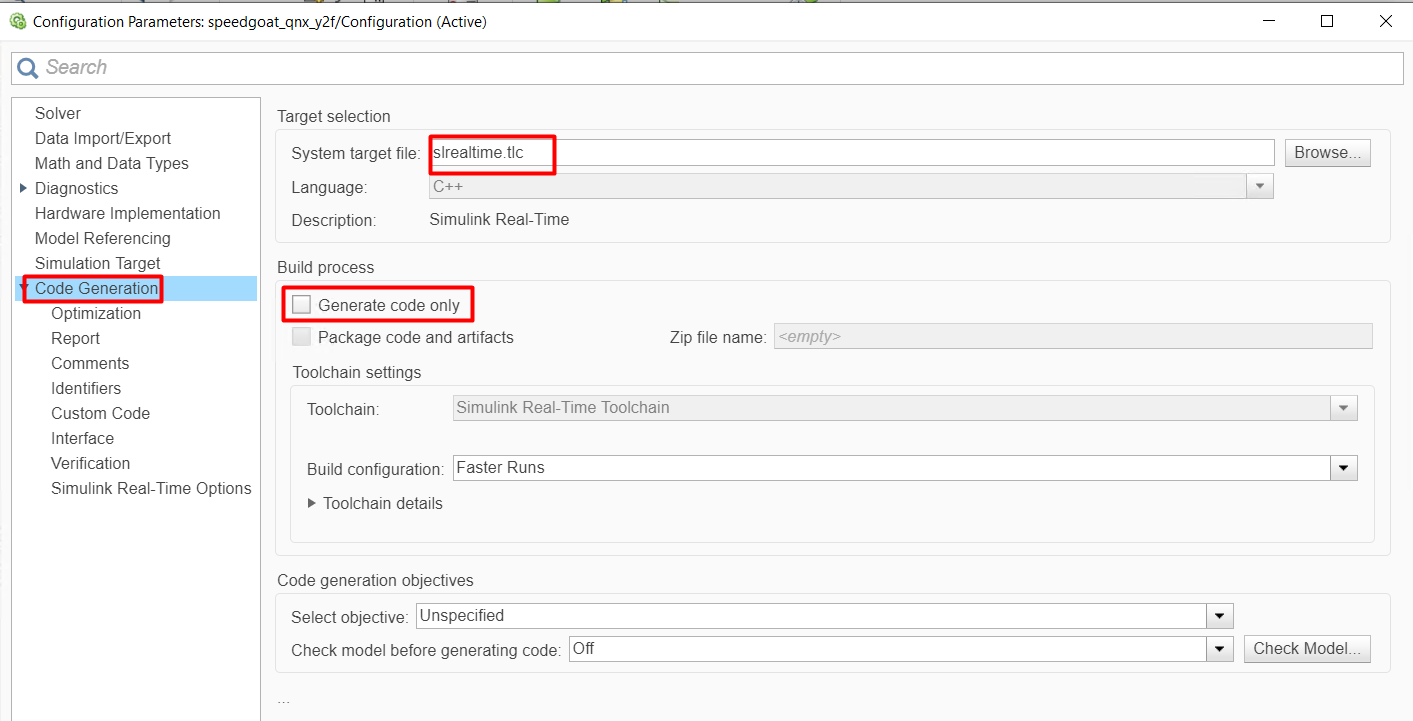Figure 13.109 Set the Simulink code generation options.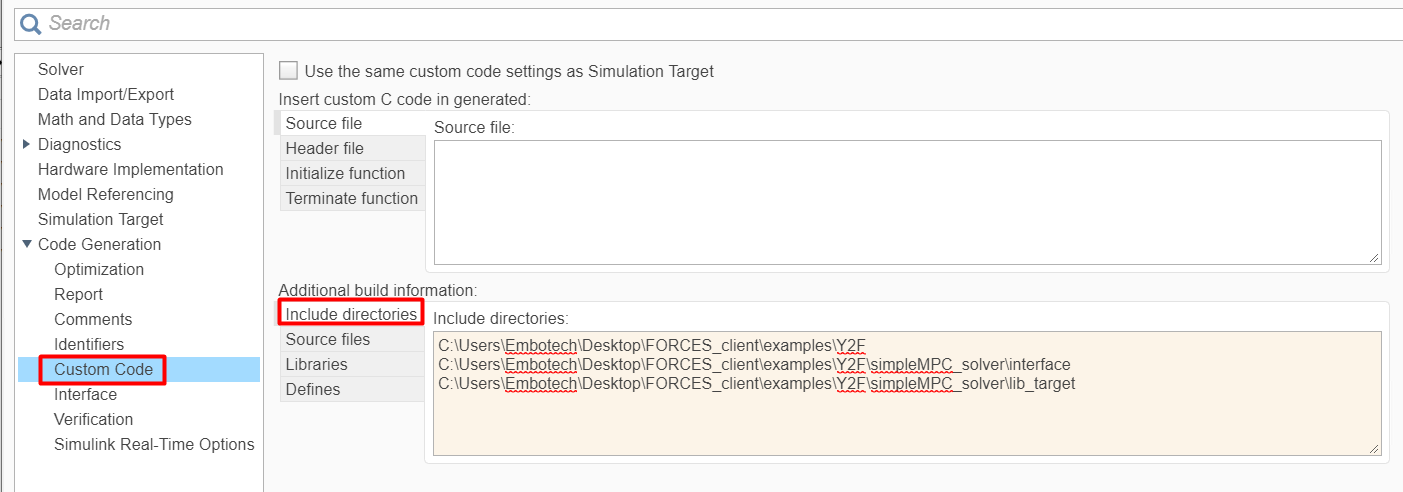Figure 13.110 Add the directories included for the code generation.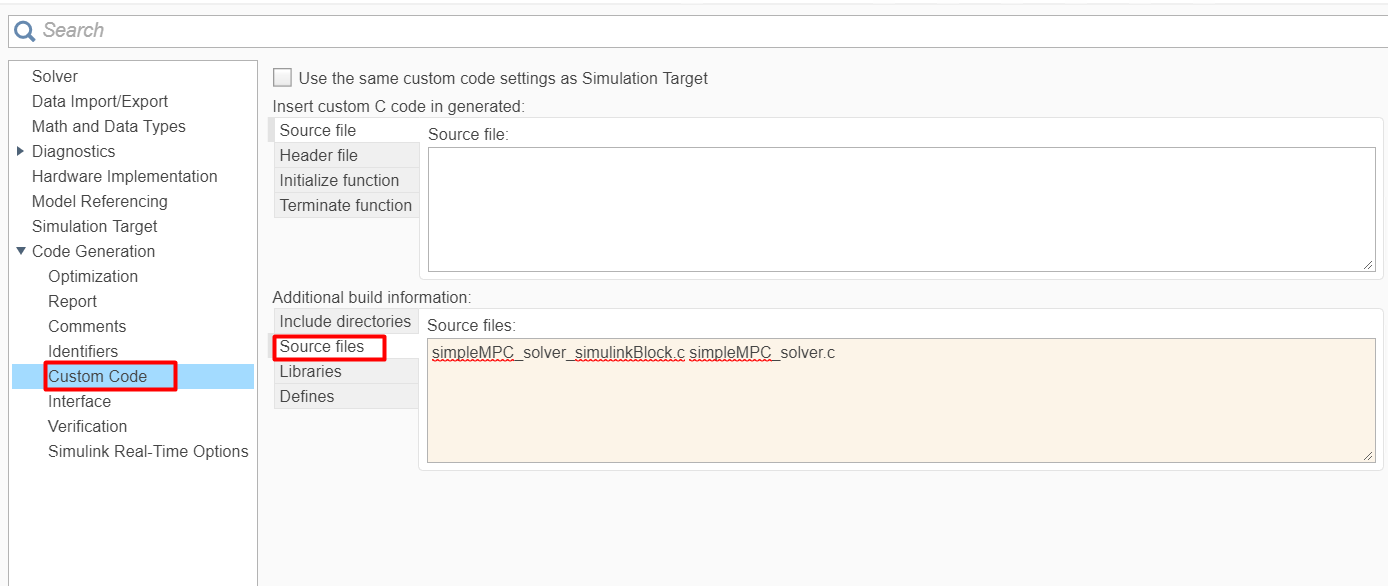Figure 13.111 Add the source files used for the code generation.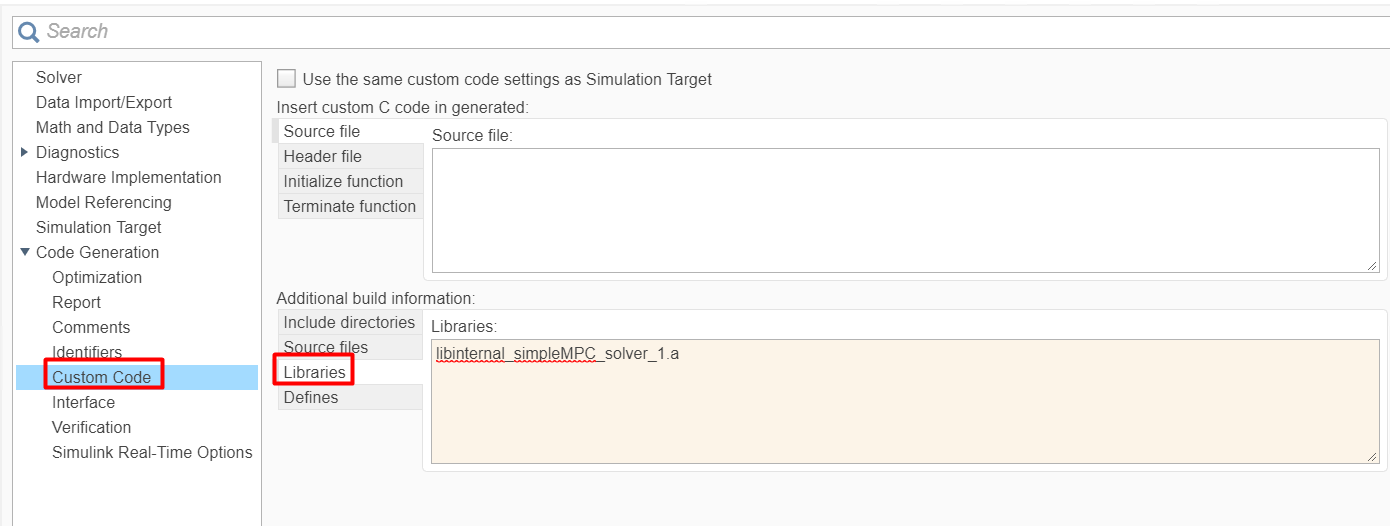Figure 13.112 Add the libraries used for the code generation.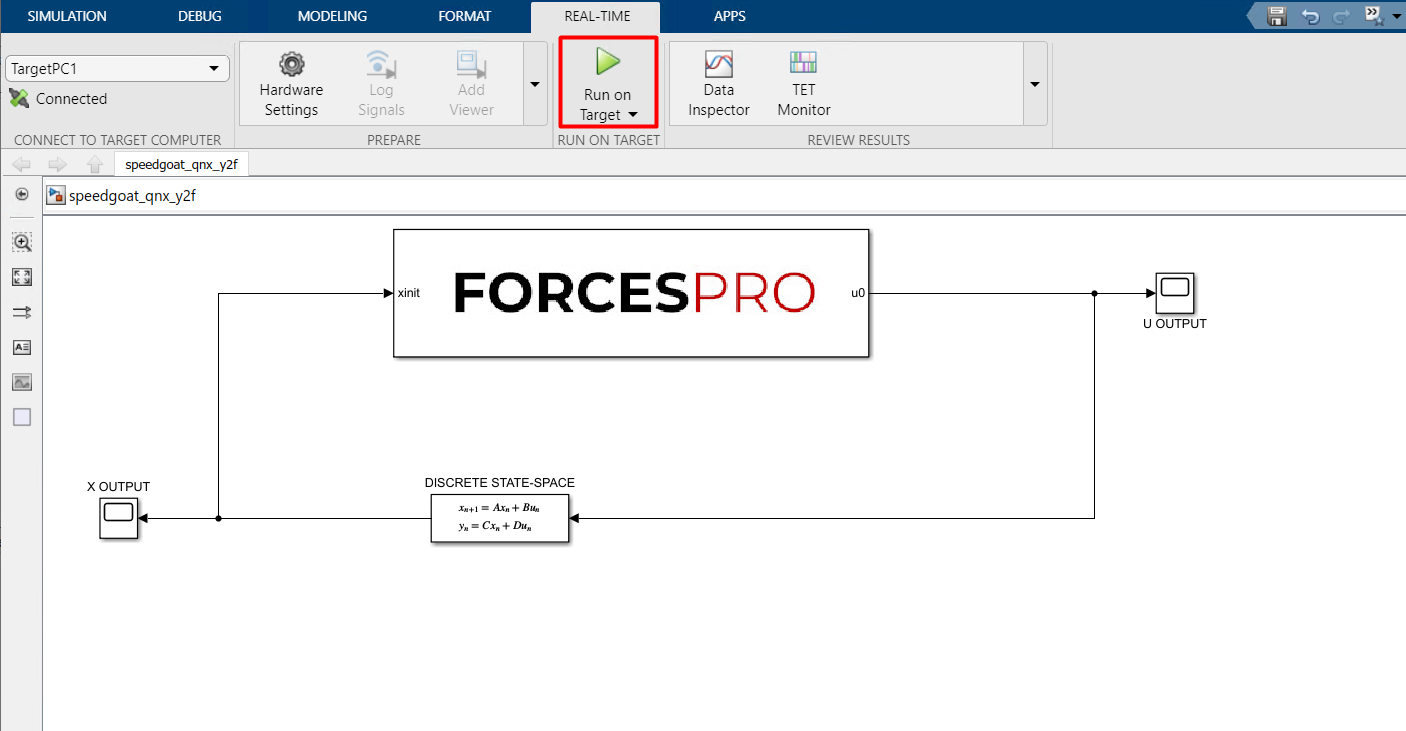Figure 13.113 Compile the code of the Simulink model.